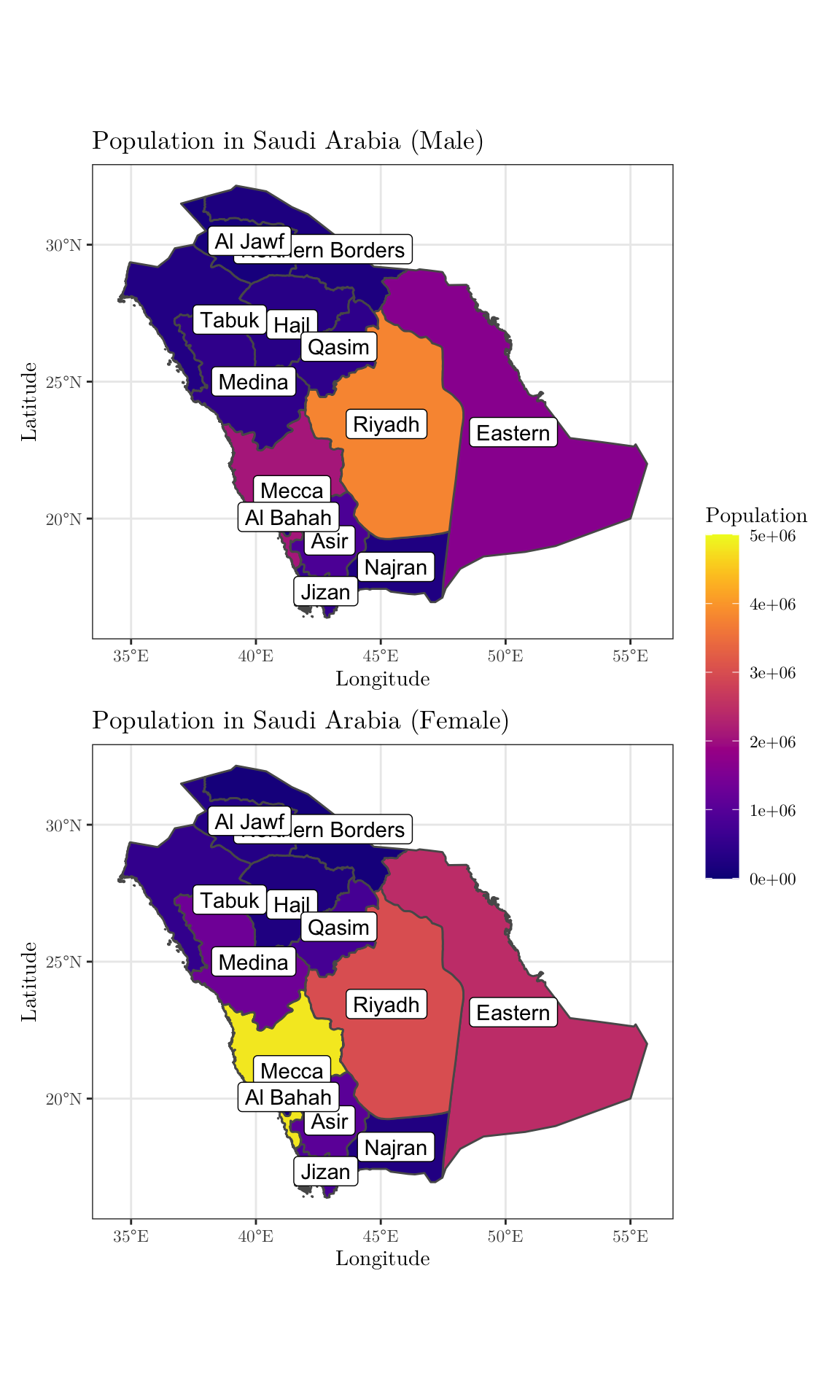# Objectives

In this tutorial session, we will see how to read, manipulate and visualize data. The main reference for this session is the R for Data Science book, which is freely available online.

# 1 Introduction

For this problem set, we will use the following libraries

library(tidyverse)
library(lubridate)
library(zoo)
library(rgeoboundaries)
library(patchwork)
library(viridis)

Throughout the tutorial, consider the following scenario. Let $$\{y_{it}; i = 1, \cdots, I; t = 1, \cdots, T\}$$ denotes a multivariate time series of disease counts in each Saudi Arabia region, such that $$I = 13$$ refers to the number of considered regions and $$T = 36$$ to the length of the time series. In particular, we will model $$Y_{it}|Y_{i,(t-1)} = y_{i,(t-1)} \sim \text{Poisson}(\lambda_{it})$$, such that $$\log(\lambda_{it}) = \beta_0 + e_{i} + \beta_1 \cdot t + \beta_2 \cdot \sin(\omega t) + \beta_3 \cdot \cos(\omega t)$$, where $$e_i$$ corresponds to the population fraction in region $$i$$ and $$\omega = 2\pi / 12$$. Also, we have information about the population size and proportion of men in each region, as well as the number of deaths linked to each element of the $$\{y_{it}\}_{it}$$ series.

You can download the .csv file here. And you read the file and convert it to a tibble object in the following way

data <- readr::read_csv(file = 'datasets/r4ds.csv')
## Rows: 468 Columns: 6
## ── Column specification ────────────────────────────────────────────────────────
## Delimiter: ","
## chr  (1): region
## dbl  (4): n_cases, pop, men_prop, n_deaths
## date (1): date
##
## ℹ Use spec() to retrieve the full column specification for this data.
## ℹ Specify the column types or set show_col_types = FALSE to quiet this message.
head(data, 5)
## # A tibble: 5 × 6
##   date       region           n_cases     pop men_prop n_deaths
##   <date>     <chr>              <dbl>   <dbl>    <dbl>    <dbl>
## 1 2018-01-01 Eastern               33 4105780    0.403        3
## 2 2018-01-01 Najran                23  505652    0.486        1
## 3 2018-01-01 Northern Borders      30  320524    0.666        6
## 4 2018-01-01 Ha’il                 36  597144    0.601        3
## 5 2018-01-01 Riyadh                38 6777146    0.559        6

The code to generated such a data set can be found here.

# 2 Data manipulation

Now, to manipulate our data set, we will use the dplyr package. From , we can see that there are 5 main methods, namely, mutate(), select(), filter(), summarise(), and arrange().

Start by selecting the date, region, and n_cases from our original data set. To do this, use the pipe operator %>% (in RStudio, you may use the shortcut Ctrl + Shift + M).

data %>% select(date, region, n_cases) %>% head(3)
## # A tibble: 3 × 3
##   date       region           n_cases
##   <date>     <chr>              <dbl>
## 1 2018-01-01 Eastern               33
## 2 2018-01-01 Najran                23
## 3 2018-01-01 Northern Borders      30
# Alternatively,
# data %>% select(-pop, -men_prop, -n_deaths) %>% head(3)
# data %>% select(date:n_cases) %>% head(3)

Using ?tidyselect::select_helpers, one may find useful functions that can be combined with select(). For instance, select all columns that start with “n_”.

data %>% select(starts_with('n_')) %>% head(3)
## # A tibble: 3 × 2
##   n_cases n_deaths
##     <dbl>    <dbl>
## 1      33        3
## 2      23        1
## 3      30        6

Aiming to obtain more meaningful sliced data sets, use the filter() and select() functions to select the the dates and regions for which the number of cases is greater than 40.

data %>% filter(n_cases > 30) %>% select(date, region) %>% head(3)
## # A tibble: 3 × 2
##   date       region
##   <date>     <chr>
## 1 2018-01-01 Eastern
## 2 2018-01-01 Ha’il
## 3 2018-01-01 Riyadh

Also, select the date, name of the region, number of cases, and number of deaths for which the region is Eastern or Najran AND the date is greater than 2019-01-01 (you may want to use the lubridate package to deal with dates).

data %>% filter(region %in% c('Eastern', 'Najran'), date > ymd('2019-01-01')) %>%
select(date, region, n_cases, n_deaths) %>% head(3)
## # A tibble: 3 × 4
##   date       region  n_cases n_deaths
##   <date>     <chr>     <dbl>    <dbl>
## 1 2019-02-01 Eastern      26        7
## 2 2019-02-01 Najran        2        0
## 3 2019-03-01 Eastern      83       13

Now, select the date, name of the region, number of cases, and number of deaths for which the number of cases is larger than 700 OR the number of deaths is equal to 10 and arrange, in a descending order for n_cases, the results.

data %>% filter(n_deaths == 10 | n_cases > 700) %>%
select(date, region, n_cases, n_deaths) %>% arrange(desc(n_cases)) %>% head(3)
## # A tibble: 3 × 4
##   date       region n_cases n_deaths
##   <date>     <chr>    <dbl>    <dbl>
## 1 2018-05-01 Mecca      792       93
## 2 2018-05-01 Riyadh     719      121
## 3 2019-05-01 Ha’il       94       10

Finally, using the mutate() function, create a new column into the original data set that shows the cumulative sum for the number of deaths (and name it cum_deaths), and select the number of deaths and this newly created column.

data %>% mutate(cum_deaths = cumsum(n_deaths)) %>%
select(ends_with('deaths')) %>% head(3)
## # A tibble: 3 × 2
##   n_deaths cum_deaths
##      <dbl>      <dbl>
## 1        3          3
## 2        1          4
## 3        6         10

Remark: cummin(), cummax(), cummean(), lag(), etc. are examples of functions that can be used with mutate().

Using the lag() and drop_na() functions, create a new column named n_cases_lag2 and another one named n_deaths_lag3 that are copies of n_cases and n_deaths but with lags 2 and 3, respectively. Then, drop the rows with NAs in the n_cases_lag2 column and select all but the date and region columns.

data %>% mutate(n_cases_lag2 = lag(n_cases, 2),
n_deaths_lag3 = lag(n_deaths, 3)) %>%
drop_na(n_cases_lag2) %>%
select(-date, -region) %>%
head(3)
## # A tibble: 3 × 6
##   n_cases     pop men_prop n_deaths n_cases_lag2 n_deaths_lag3
##     <dbl>   <dbl>    <dbl>    <dbl>        <dbl>         <dbl>
## 1      30  320524    0.666        6           33            NA
## 2      36  597144    0.601        3           23             3
## 3      38 6777146    0.559        6           30             1

Now, the goal is work with the group_by() function. To do this, group the data set by region, and select the three first columns.

data %>% group_by(region) %>% select(1:3) %>% head(3)
## # A tibble: 3 × 3
## # Groups:   region 
##   date       region           n_cases
##   <date>     <chr>              <dbl>
## 1 2018-01-01 Eastern               33
## 2 2018-01-01 Najran                23
## 3 2018-01-01 Northern Borders      30

Combining a few different functions, select all but the pop column and create a new one (named norm_cases) that shows the normalized number of cases by date.

data %>% group_by(date) %>%
mutate(norm_cases = n_cases/sum(n_cases),
cumsum = cumsum(norm_cases)) %>%
select(-pop) %>%
head(14)
## # A tibble: 14 × 7
## # Groups:   date 
##    date       region           n_cases men_prop n_deaths norm_cases cumsum
##    <date>     <chr>              <dbl>    <dbl>    <dbl>      <dbl>  <dbl>
##  1 2018-01-01 Eastern               33    0.403        3     0.0862 0.0862
##  2 2018-01-01 Najran                23    0.486        1     0.0601 0.146
##  3 2018-01-01 Northern Borders      30    0.666        6     0.0783 0.225
##  4 2018-01-01 Ha’il                 36    0.601        3     0.0940 0.319
##  5 2018-01-01 Riyadh                38    0.559        6     0.0992 0.418
##  6 2018-01-01 Asir                  21    0.444        4     0.0548 0.473
##  7 2018-01-01 Mecca                 40    0.302        9     0.104  0.577
##  8 2018-01-01 Tabuk                 33    0.347        7     0.0862 0.663
##  9 2018-01-01 Medina                29    0.263        3     0.0757 0.739
## 10 2018-01-01 Qasim                 14    0.373        2     0.0366 0.775
## 11 2018-01-01 Al Bahah              34    0.593        9     0.0888 0.864
## 12 2018-01-01 Jizan                 33    0.386        4     0.0862 0.950
## 13 2018-01-01 Al Jawf               19    0.298        3     0.0496 1
## 14 2018-02-01 Eastern               67    0.403       10     0.0860 0.0860

Using the summarise() (or summarize()) function, get the average and variance for the variables n_cases and n_deaths, as well as the total number of rows.

data %>% select(n_cases, n_deaths) %>%
summarize(mean_n_cases = mean(n_cases, na.rm = TRUE),
var_n_cases = var(n_cases, na.rm = TRUE),
mean_n_deaths = mean(n_deaths, na.rm = TRUE),
var_n_deaths = var(n_deaths, na.rm = TRUE), n = n())
## # A tibble: 1 × 5
##   mean_n_cases var_n_cases mean_n_deaths var_n_deaths     n
##          <dbl>       <dbl>         <dbl>        <dbl> <int>
## 1         63.9      11830.          9.76         294.   468

Notice that the result from the above item is not very impressive, but we can combine it with group_by() to get more meaningful statistics. For instance, obtain similar results as before, but for each region.

data %>% group_by(region) %>%
select(n_cases, n_deaths) %>%
summarize(mean_n_cases = mean(n_cases, na.rm = TRUE),
var_n_cases = var(n_cases, na.rm = TRUE),
mean_n_deaths = mean(n_deaths, na.rm = TRUE),
var_n_deaths = var(n_deaths, na.rm = TRUE), n = n())
## Adding missing grouping variables: region
## # A tibble: 13 × 6
##    region           mean_n_cases var_n_cases mean_n_deaths var_n_deaths     n
##    <chr>                   <dbl>       <dbl>         <dbl>        <dbl> <int>
##  1 Al Bahah                 39.7       4043.          7.44        221.     36
##  2 Al Jawf                  39.9       2953.          5.83         91.8    36
##  3 Asir                     54.5       4383.          8.94        142.     36
##  4 Eastern                  84.2      11258.         12.6         245.     36
##  5 Ha’il                    47.3       4685.          8.58        258.     36
##  6 Jizan                    55.0       5881.          9.39        236.     36
##  7 Mecca                   185.       49857.         26.9        1150.     36
##  8 Medina                   49.0       6594.          6.81        141.     36
##  9 Najran                   33.3       2260           5.56         78.4    36
## 10 Northern Borders         36.6       3947.          5.58        108.     36
## 11 Qasim                    37.6       3111.          5.28         65.2    36
## 12 Riyadh                  126.       30390.         17.1         600.     36
## 13 Tabuk                    42.4       4215.          6.92        119.     36

And as in SQL, in tidyverse, there are also functions that make joining two tibbles possible. From the documentation page, we have inner_join(), left_join, right_join() and full_join(). As an example, consider the following data set, which shows the (fake) mean temperature (in Celsius) in almost all regions and studied months in Saudi Arabia. Assuming the previous data set is in data,

set.seed(0)
n <- (nrow(data) - 3)
new_data <- tibble(id = 1:n, date = data$date[1:n], region = data$region[1:n], temperature = round(rnorm(n, 30, 2), 1))
new_data
## # A tibble: 465 × 4
##       id date       region           temperature
##    <int> <date>     <chr>                  <dbl>
##  1     1 2018-01-01 Eastern                 32.5
##  2     2 2018-01-01 Najran                  29.3
##  3     3 2018-01-01 Northern Borders        32.7
##  4     4 2018-01-01 Ha’il                   32.5
##  5     5 2018-01-01 Riyadh                  30.8
##  6     6 2018-01-01 Asir                    26.9
##  7     7 2018-01-01 Mecca                   28.1
##  8     8 2018-01-01 Tabuk                   29.4
##  9     9 2018-01-01 Medina                  30
## 10    10 2018-01-01 Qasim                   34.8
## # … with 455 more rows

Notice that there is a column named id on new_data. We will need it to link the new tibble to the old one. Thus, let’s create a similar column in data.

data <- data %>% add_column(id = 1:(nrow(data))) %>% select(c(7, 1:6))
head(data, 1)
## # A tibble: 1 × 7
##      id date       region  n_cases     pop men_prop n_deaths
##   <int> <date>     <chr>     <dbl>   <dbl>    <dbl>    <dbl>
## 1     1 2018-01-01 Eastern      33 4105780    0.403        3

Now, we can incorporate the new data into the original tibble by using, for example, the left_join() function.

data %>% left_join(new_data %>% select(id, temperature), by = 'id') %>%
tail(1)
## # A tibble: 1 × 8
##      id date       region  n_cases    pop men_prop n_deaths temperature
##   <int> <date>     <chr>     <dbl>  <dbl>    <dbl>    <dbl>       <dbl>
## 1   468 2020-12-01 Al Jawf       2 440009    0.298        0          NA

# 3 Data visualization

For this section, we will use ggplot2 to produce different plots.

## 3.1 Data preparation

From the original data set, create an object that contains the columns date, region, n_men, n_women, n_cases, and n_deaths, such that n_men and n_women correspond to the number of men and the number of women, respectively (to do this, recall that men_prop represents the proportion of men in the population for a given region).

data <- data %>% mutate(women_prop = 1 - men_prop) %>%
mutate(n_men = pop * men_prop) %>%
mutate(n_women = pop * women_prop) %>%
select(date, region, n_men, n_women, n_cases, n_deaths)
head(data, 7)
## # A tibble: 7 × 6
##   date       region              n_men  n_women n_cases n_deaths
##   <date>     <chr>               <dbl>    <dbl>   <dbl>    <dbl>
## 1 2018-01-01 Eastern          1654276. 2451504.      33        3
## 2 2018-01-01 Najran            245548.  260104.      23        1
## 3 2018-01-01 Northern Borders  213622.  106902.      30        6
## 4 2018-01-01 Ha’il             358851.  238293.      36        3
## 5 2018-01-01 Riyadh           3785117. 2992029.      38        6
## 6 2018-01-01 Asir              850468. 1062924.      21        4
## 7 2018-01-01 Mecca            2086623. 4828383.      40        9

## 3.2 Plotting

Now, the main goal is visualize the studied data set. To do this, we will mainly rely on the ggplot2 library, which is also included in the tidyverse universe.

Start by creating a line chart for the number of cases in each region (all in the same plot) over the months (when I tried to plot it in my RMarkdown document, I had problems with the Ha’il name. If the same happens to you, try to replace it by Hail). Map region to color.

data <- data %>% mutate(region = replace(region, region == 'Ha’il', 'Hail'))
ggplot(data = data,
mapping = aes(x = date, y = n_cases, color = region)) +
geom_line() +
theme_bw() +
labs(x = 'Date', y = 'Number of Cases', color = 'Region') +
theme(text = element_text(family = 'LM Roman 10'))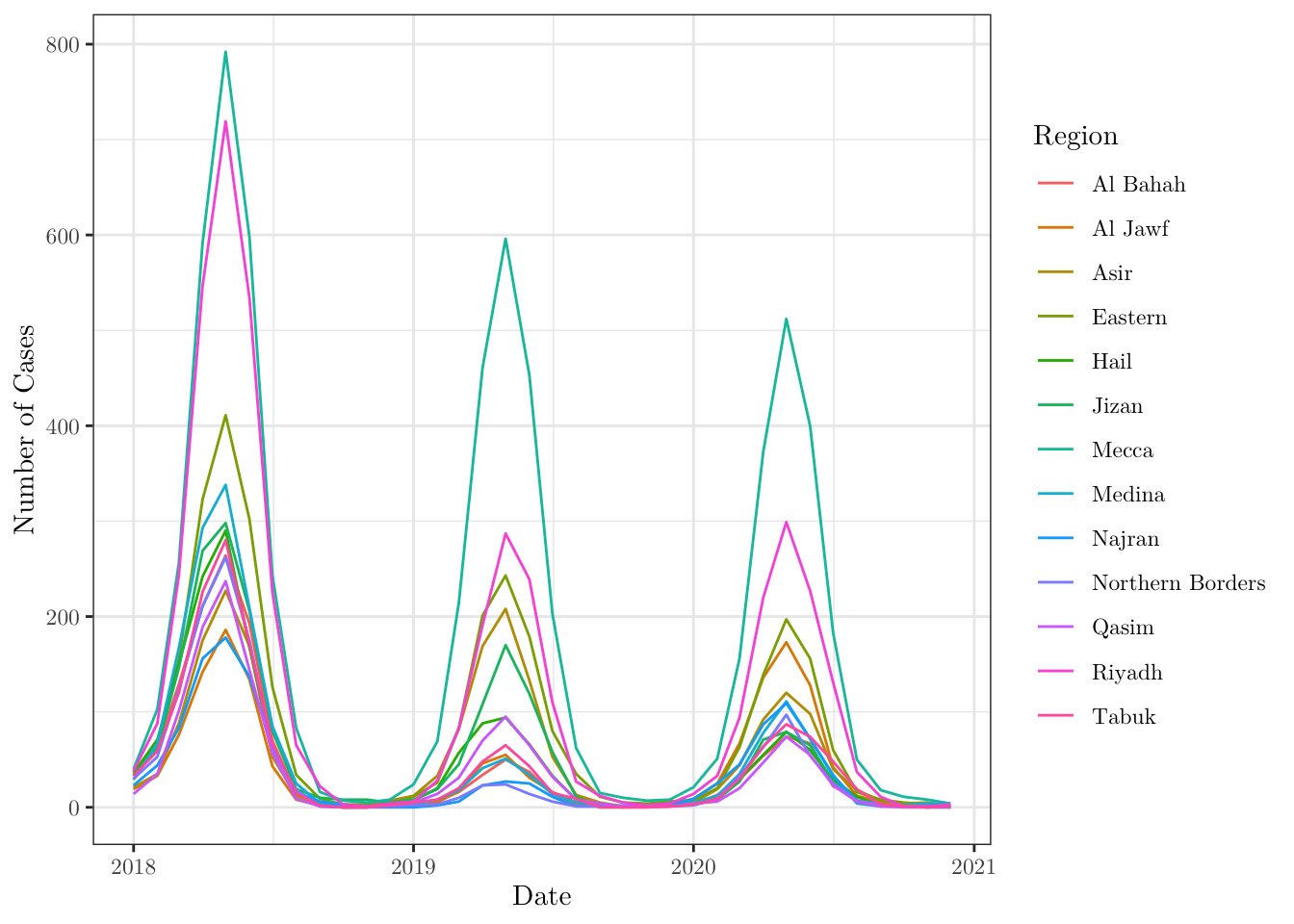When plotting, we can also map more than one property at a time. For instance, plot a dot chart for the number of cases in each region over months that maps region to color and n_deaths to size.

ggplot(data = data,
mapping = aes(x = date, y = n_cases, size = n_deaths, color = region)) +
geom_point() +
theme_bw() +
labs(x = 'Date', y = 'Number of Cases', color = 'Region', size = 'N. Deaths') +
theme(text = element_text(family = 'LM Roman 10'))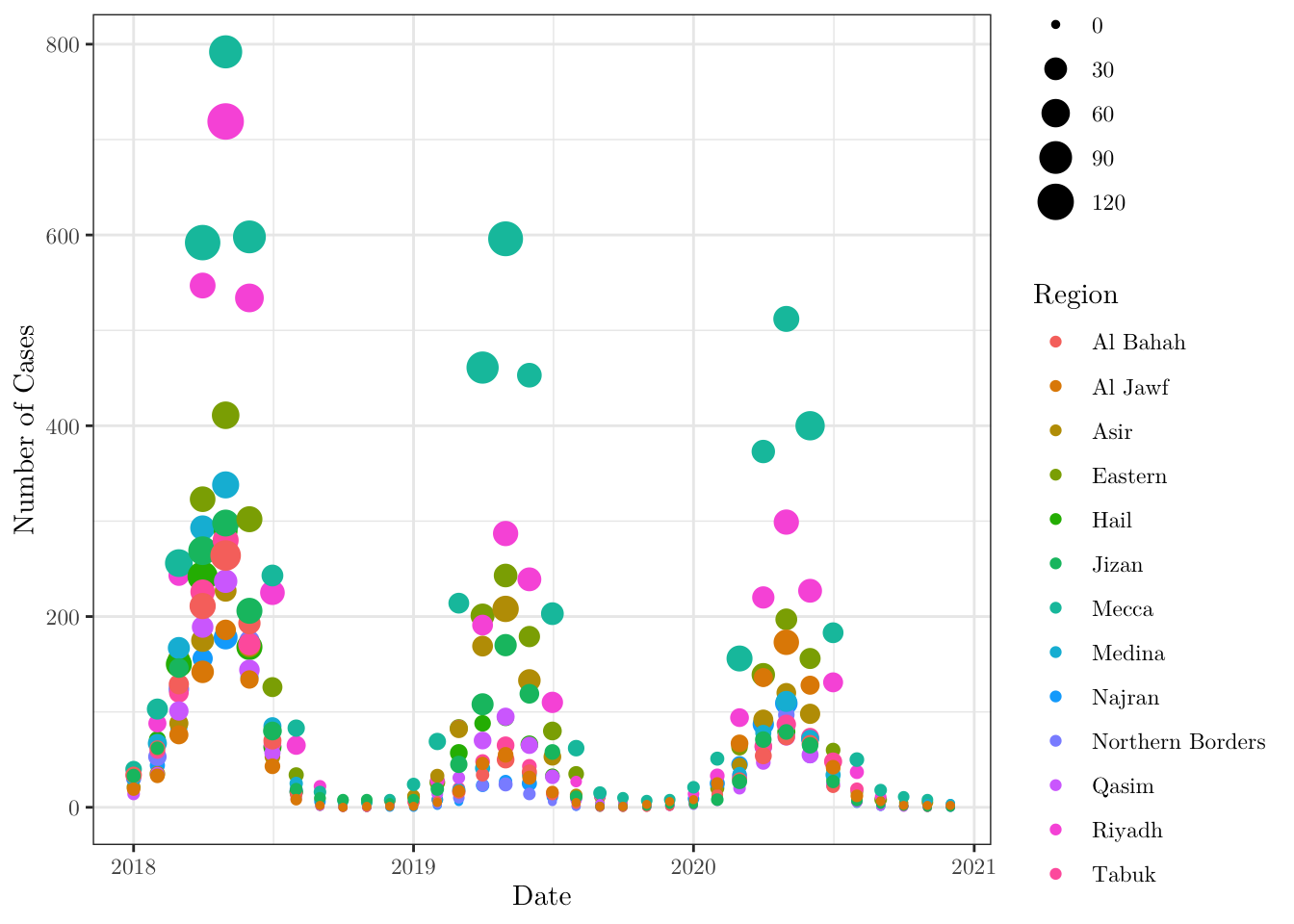Now, using the function rollmean() from the zoo package, plot the number of cases in Mecca (notice that you will have to filter your data set) over time and the moving average (with window $$\text{k} = 3$$) of the same time series.

ggplot(data = data,
mapping = aes(x = date, y = n_cases)) +
geom_line(data = . %>% filter(region == 'Mecca'), aes(color = 'light_red')) +
geom_line(data = . %>% filter(region == 'Mecca'),
aes(y = rollmean(x = n_cases,
k = 3,
align = 'right',
fill = NA),
color = 'solid_red')) +
theme_bw() +
labs(x = 'Date', y = 'Number of Cases',
title = 'Number of cases in Mecca (with moving average for k = 3)') +
scale_color_manual(name = 'Number of cases (Mecca)',
values = c('light_red' = alpha('red', 0.25),
'solid_red' = alpha('red', 1.00)),
labels = c('Original data', 'Moving average')) +
theme(text = element_text(family = 'LM Roman 10'))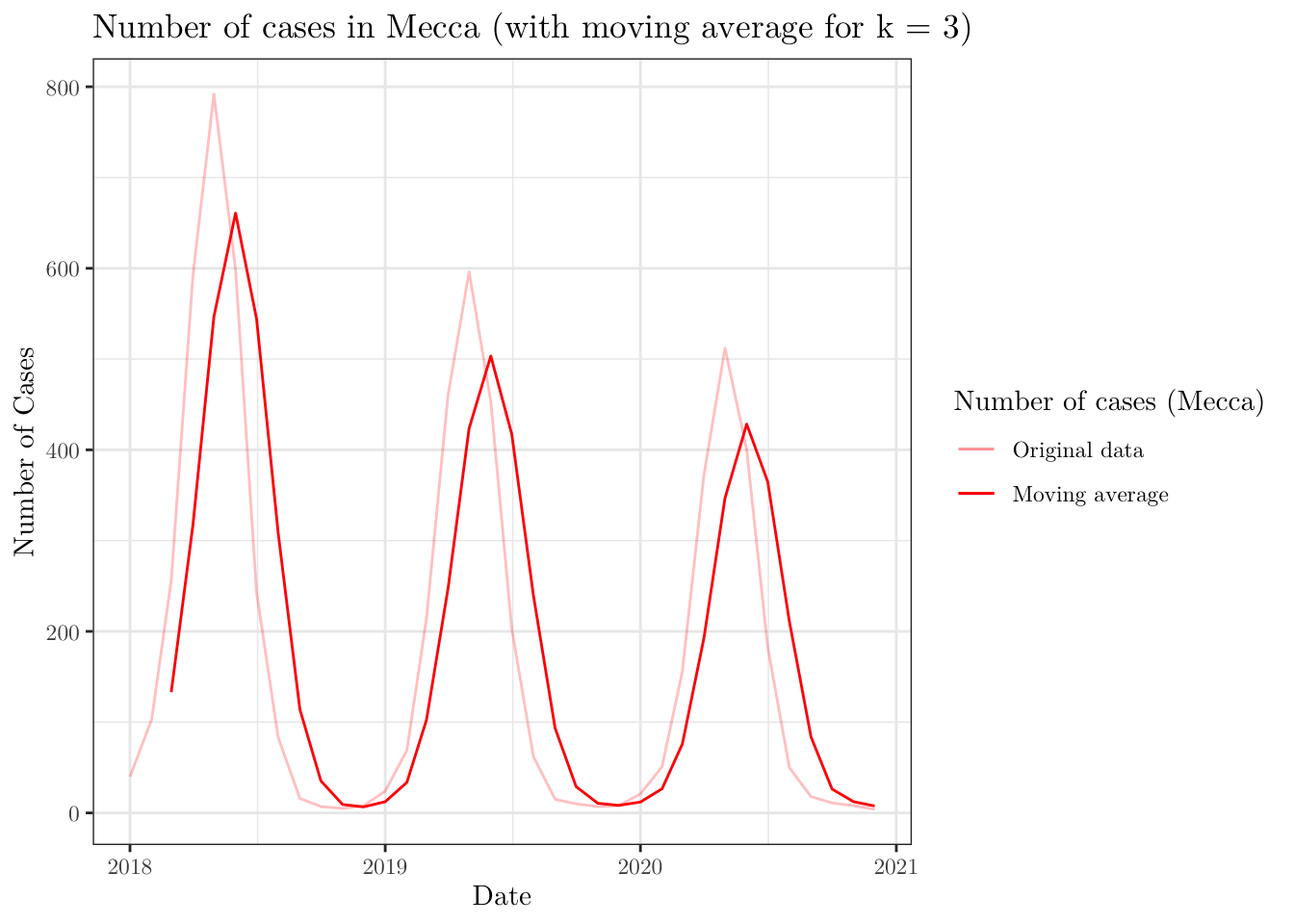Now, we will plot the map of Saudi Arabia divided into the given regions. To do this, we will need to work with a shapefile for the boundaries of the Kingdom. This type of file may be found online. However, there are some R packages that provide such data. For instance, we can use the geoboundaries('Saudi Arabia', adm\_lvl = 'adm1') function from the rgeoboundaries package to obtain the desired data set.

After downloading the data, you can plot it using the ggplot(data) + geom_sf() functions (depending on what you are trying to do, you may have to install (and load) the sp and/or sf libraries).

KSA_shape <- geoboundaries('Saudi Arabia', adm_lvl = 'adm1')
KSA_shape$shapeName <- data$region[1:13]

ggplot(data = KSA_shape) +
geom_sf() +
geom_sf_label(aes(label = shapeName)) +
theme_bw() +
labs(x = 'Longitude', y = 'Latitude', title = 'Map of Saudi Arabia') +
theme(text = element_text(family = 'LM Roman 10'))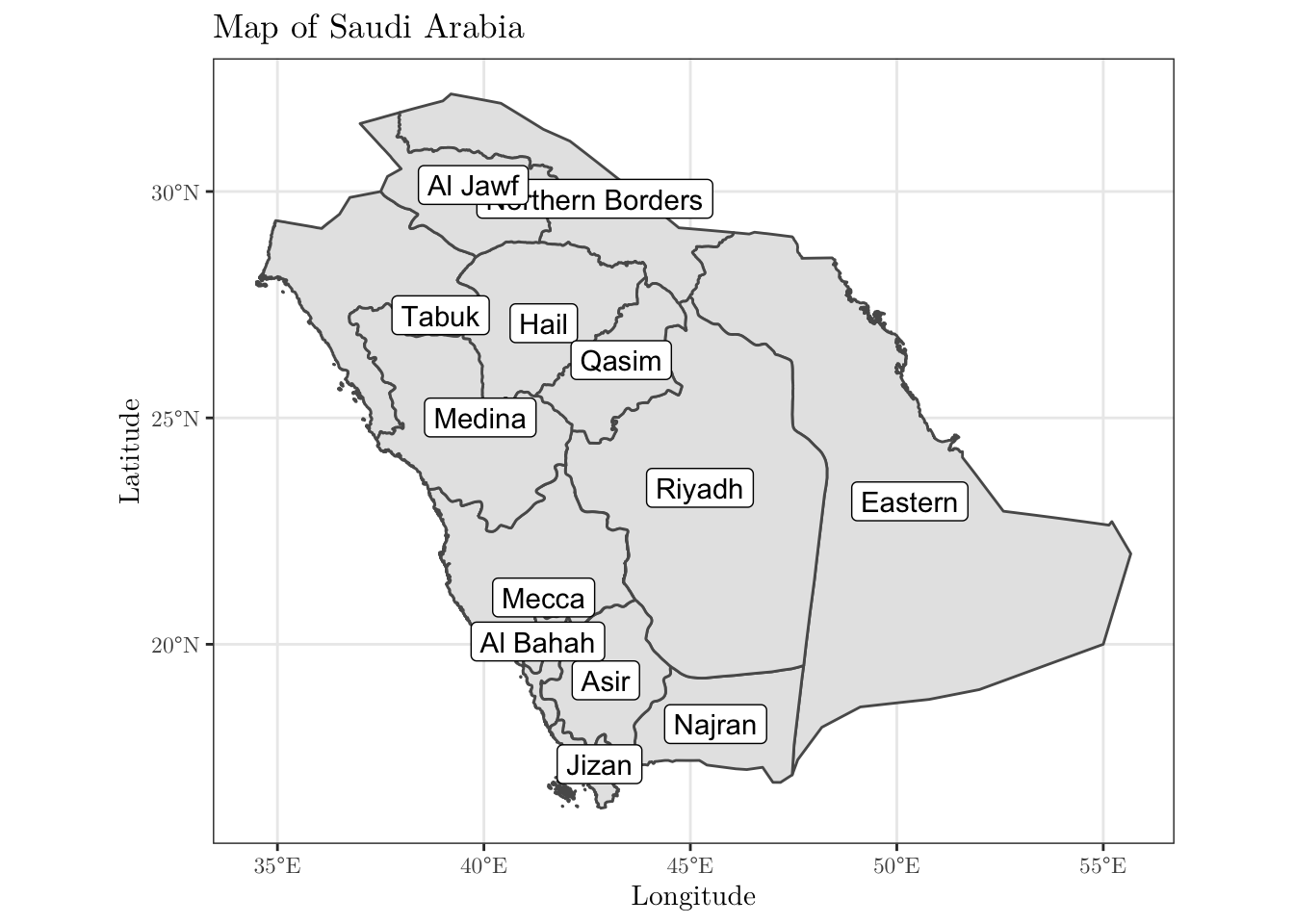As a final task, plot two versions of the above map side-by-side. On the left, the colors should represent the population size for men in each region; similarly, on the right, the population size for women (to combine plots in this way, you may want to use the patchwork package. And for different colour palettes, you may use the viridis package). Use the same scale, so that the values are comparable.

KSA_shape$n_men <- data$n_men[1:13]
KSA_shape$n_women <- data$n_women[1:13]

min_pop = 0      # min(c(KSA_shape$n_men, KSA_shape$n_women))
max_pop = 5e+06  # max(c(KSA_shape$n_men, KSA_shape$n_women))

men <- ggplot(data = KSA_shape) +
geom_sf(aes(fill = n_men)) +
scale_fill_viridis(limits = c(min_pop, max_pop), name = 'Population',
option = 'plasma') +
geom_sf_label(aes(label = shapeName)) +
theme_bw() +
labs(x = 'Longitude', y = 'Latitude',
title = 'Population in Saudi Arabia (Male)') +
theme(text = element_text(family = 'LM Roman 10'))

women <- ggplot(data = KSA_shape) +
geom_sf(aes(fill = n_women)) +
scale_fill_viridis(limits = c(min_pop, max_pop), name = 'Population',
option = 'plasma') +
geom_sf_label(aes(label = shapeName)) +
theme_bw() +
labs(x = 'Longitude', y = 'Latitude',
title = 'Population in Saudi Arabia (Female)') +
theme(text = element_text(family = 'LM Roman 10'))

combined <- men + women  + plot_layout(guides = 'collect', ncol = 1) &
theme(legend.position = 'right', legend.key.height = unit(1.25, 'cm'))

print(combined)